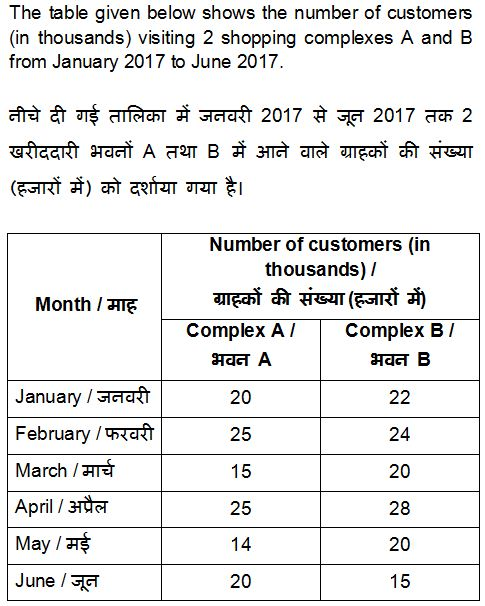# SSC MTS 2016 Re-Exam Paper : Held on 09-OCT-2017 Shift-1 (Numerical Aptitude)

QID : 26 - If a number is divided by 30 then it leaves 17 as a remainder. What will be the remainder when the same number is divided by 10?
Options:
1) 7
2) 3
3) 1
4) 2
QID : 27 - What is the value of 162 + 172 + 182 + ……. 252?
Options:
1) 4325
2) 4465
3) 4105
4) 4285
QID : 28 - How many times digit '5' appears in the number from 1 to 100?
Options:
1) 20
2) 21
3) 19
4) 18
QID : 29 - What is the unit digit of 5124 x 1245?
Options:
1) 5
2) 1
3) 0
4) 2
QID : 30 - What is the value of [(a–2b3) ÷ (a1b–1)] x [(a2b–4) ÷ (a–1b2)]?
Options:
1) b2
2) 1/b2
3) a2
4) a2b2
QID : 31 - Which of the following number is divisible by 11?
Options:
1) 59609
2) 45332
3) 23581
4) 44433
QID : 32 - A can do a piece of work in 20 days and B can do the same piece of work in 30 days. They start working together and work for 5 days and then both leave the work. C alone finishes the remaining work in 14 days. In how many days will C alone finish the whole work?
Options:
1) 24
2) 18
3) 36
4) 42
QID : 33 - P, Q and R undertook a work for Rs 48000. Together P and Q complete 5/12th part of the work. What is the share (in Rs) of R?
Options:
1) 21000
2) 28000
3) 27000
4) 31000
QID : 34 - The length of two parallel sides of a trapezium are 30 cm and 40 cm. If the area of the trapezium is 350 cm2, then what is the value (in cm) of its height?
Options:
1) 8
2) 10
3) 15
4) 12
QID : 35 - If the difference between discount of 35% and two successive discounts of 20% on a certain bill is Rs 3, then what is the amount (in Rs) of the bill?
Options:
1) 250
2) 300
3) 350
4) 400
QID : 36 - If a shopkeeper marks the price of goods 40% more than their cost price and allows a discount of 40%, then what is his gain or loss percent?
Options:
1) 16%, Loss
2) 16%, Profit
3) 10%, Loss
4) 12%, Profit
QID : 37 - The ratio of two positive numbers is 9 : 11. Their product is 6336. What is the smallest number?
Options:
1) 32
2) 72
3) 88
4) 48
QID : 38 - B starts some business by investing Rs 90000. After 4 months, D joins business by investing Rs 80000. At the end of the year, in what ratio will they share the profit?
Options:
1) 10 : 7
2) 9 : 4
3) 27 : 16
4) 7 : 3
QID : 39 - Average of 9 numbers is 20. If a number 30 is also included, then what will be the average of these 10 numbers?
Options:
1) 20.5
2) 21
3) 19.5
4) 21.5
QID : 40 - By selling 50 metres of cloth, a person gains the cost price of 20 metres of cloth. What is his gain percent?
Options:
1) 40
2) 25
3) 20
4) 10

QID : 41 - If some articles are bought at Rs 10 each and sold at Rs 7 each, then what is the loss percentage?
Options:
1) 60
2) 16.67
3) 25
4) 30
QID : 42 - A man saves 30% of his income in 1 year. If he wants to save the same amount in 8 months, then by how much percentage should he increase his monthly savings?
Options:
1) 20
2) 30
3) 40
4) 50
QID : 43 - A man spends 80% of his income and saves the rest. If his income and spending both increases by 10%, then what is the percentage change in his savings?
Options:
1) 10% increase
2) 5% decrease
3) 5% increase
4) 15% decrease
QID : 44 - A car travels at a speed of 25 m/s for 8 hours. What is the distance (in km) travelled by the car?
Options:
1) 360
2) 720
3) 450
4) 900
QID : 45 - A 450 meter long train crosses a bridge 650 meters long in 36 seconds. What is speed (in km/hr) of the train?
Options:
1) 110
2) 125
3) 150
4) 95
QID : 46 - The population of a town increases at the rate of 15% per annum. If the present population is 108445 of town, then what was the population 2 years ago?
Options:
1) 72000
2) 79000
3) 82000
4) 85000
QID : 47 -What was the percentage change in number of customers to complex B from March to April?
Options:
1) 20
2) 40
3) 28
4) 56
QID : 48 -What is the maximum difference (in thousands) between the numbers of customers in the 2 complexes among the given months?
Options:
1) 5
2) 6
3) 8
4) 4
QID : 49 -What is the total number of customers (in thousands) in the 2 complexes in the month of April?
Options:
1) 52
2) 53
3) 50
4) 56